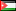##### The Relationship Between Selected Anthropometric Measures and Body Composition of Volleyball Players
Publication Type
Original research
Authors
Fulltext

The Relationship Between Selected Anthropometric Measures and Body Composition of Volleyball Players

https://journals.ju.edu.jo/DirasatEdu/article/view/655

The purpose of this study was to investigate the relationship between selected anthropometric measures and Body Composition (BC) of volleyball players. The sample consisted of (84) players, from different divisions of volleyball clubs in Palestine.The means of age, body mass and height were (24.35 years, 80.88 kg, and 1.84 m), respectively.The researcher conducted anthropometric measures (age, height, body mass, circumference of neck, biceps, hand wrist, abdomen, thigh and calf ) Body Mass Index (BMI), Body Surface Area (BSA), and three skin folds measures (chest, abdomen, thigh) to determine body density using Jackson and Pollock (1978) equation.

The results indicated that the means of circumferences of neck, biceps, hand wrist, abdomen, thigh and calf, were respectively (38.4, 30.42, 18.60, 81.60, 59.85, 38.71) centimeters, respectively. In addition, the means of percent of body fat, Lean Body Mass (LBM), Body Density (BD), (BMI), and (BSA) were (13.5%, 64.52 kg, 1.06 g/ml, 23.66 kg/m2, 2.04 m2) respectively. Also, the results revealed that the best correlation between anthropometric measures and (BC) was between fat and abdomen circumference (0.79), and between body mass and (LBM) (0.77).

Furthermore, the results of stepwise regression R2 revealed the following two equations:

%Fat =(34.949)+((0.490)*(abdomen circumference, cm))+((0.584)*(age, yr))

+((-1.590)*(hand wrist circumference, cm))+((0.294)*(body mass, kg)

(R2   =0.755)

(LBM) kg =(25.754)+((0.851)*(body mass, kg)) +((-0.606)*(abdomen circumference, cm))+((-0.686)*

(age, yr)) +((1.942)*(hand wrist circumference, cm))

(R2   =0.786)

Based on the study findings, the researcher recommended to apply these two equations for predicting (BC), the first one for fat and the other for (LBM).

Keywords:Anthropometric Measures, Body Composition, Volleyball

Full Text:PDF

Journal
Title
عبدالناصر القدومي
Publisher
مجلة دراسات (العلوم التربوية)، الجامعة الأردنية (2006)، 33(1)، 242-258.
Publisher Country
JordanPublication Type
Both (Printed and Online)
Volume
33
Year
2006
Pages
242-258